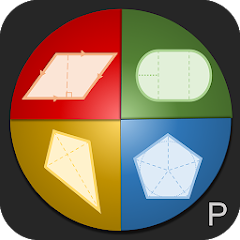# GeoCal - Geometry Calculator

500+Everyone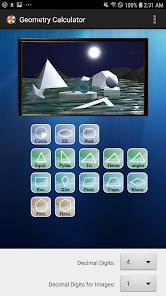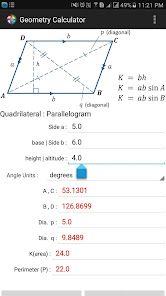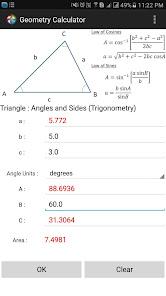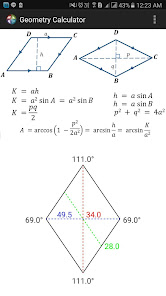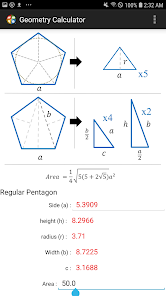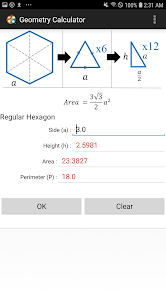With this app, you can obtain the remaining parameters of geometry shapes based on your given input. An image of a shape will also be illustrated based on your input.

Currently support 2D shapes:
Circle
Ellipse (Oval)
Triangle : Equilateral Triangle
Triangle : Pythagorean
Triangle : Area (basic formula)
Triangle : Area by sides (Heron's formula)
Triangle : Angles and Sides (Trigonometry)
Pentagon
Hexagon

Text Colors:
(Label) Blue : required input
(Textbox) Black : Input given by the user
(Textbox) Red : Output
(Textbox) Magenta : Input automatically filled up by given input